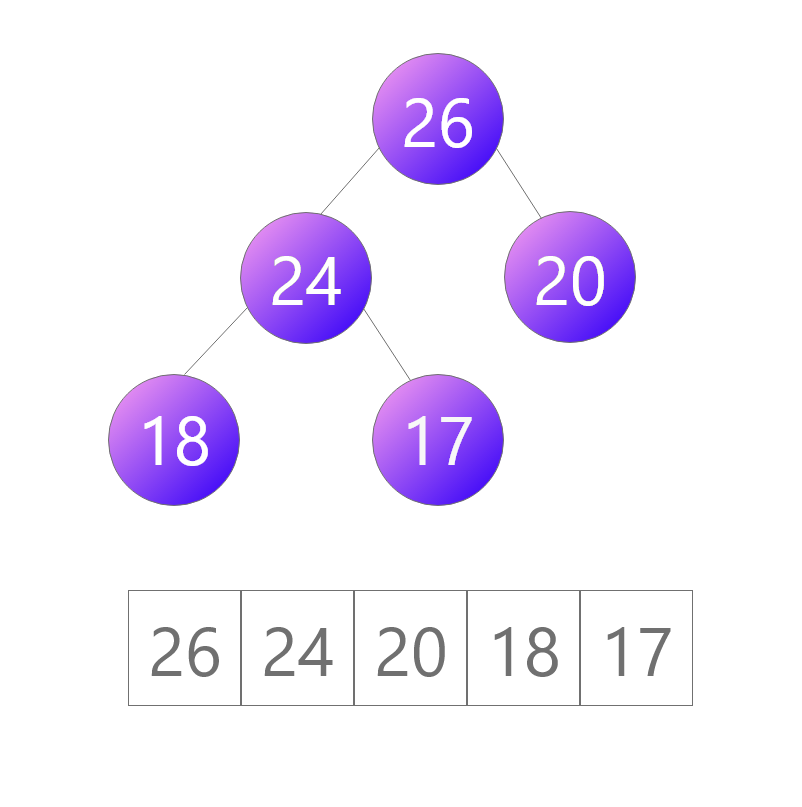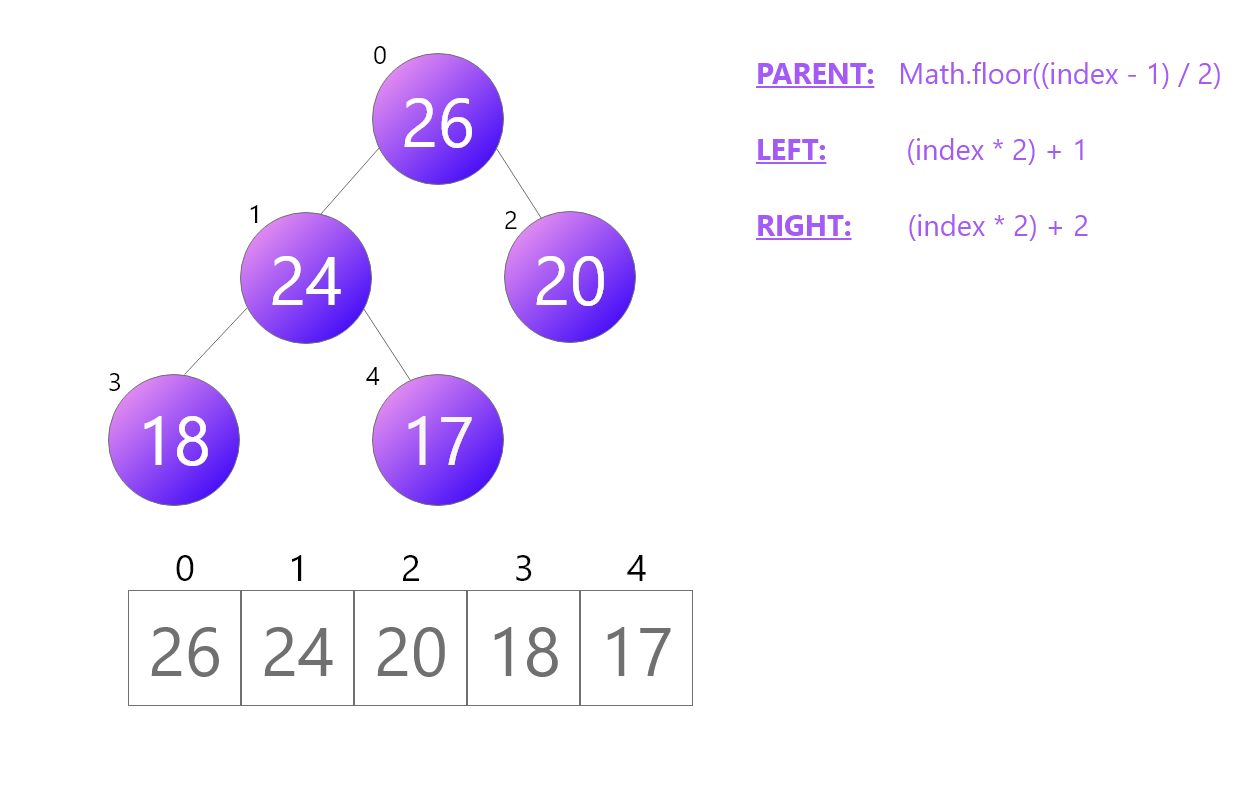# How to Create a Priority Queue With JavaScript

A priority queue is a special type of queue, where the elements within the queue have a specified priority-level.

In a traditional queue, there is a First-In-First-Out (FIFO) rule, similar to queuing up in a shop. A priority queue would enable someone with a higher priority to jump the queue and go all the way to the front of the line.

### Heaps

Heaps are a tree-based data structure, usually implemented as an array, which represent a priority queue. There are two types of heaps:

1. Min Heap
2. Max Heap

Once you know one of these, it is simple to know the other, as it is just the reverse.

In this article we will be looking at max-heaps. Afterwards, it would be a good exercise to see if you could implement a min-heap using the knowledge you have gained from this article.In the image above, you will see we have a sorted array, which can be represented as a binary tree, with the elements: 26, 24, 20, 18 & 17

Notice how each element is added to the tree from left to right. If a new element was to be inserted, it would become the left child of the node which has a priority level of 20, assuming the element had a lesser priority than this.

The first node, 26, is called the root node, in a max-heap this is the largest number, i.e. the node with the highest priority which should be extracted next. Each node can have a left child, and a right child. Notice how the children of each node are lesser than the priority value of the parent node. Each node, other than the root node, has a parent node, which is just one element up from the node you are looking at.

Elements with any priority value can be inserted in to the heap. With every insertion, there is an ordering completed to correctly position the newly inserted element.

Elements are dequeued/extracted from the root, which similar to insertion, is also followed by an ordering operation, in order to correctly position the next element with the highest priority at the root of the tree.

### Terminology

• Node: An element within the tree.
• Branch: The edges which connect the nodes together.
• Root: The top level node. This is the element with the largest value in a max-heap, and the element with the lowest value in a min-heap.
• Children: Each node can have up to 2 children; a left child, and a right child. Both which should be lower in value than their parent.
• Parent: If you follow the branch up from a node one-level, you will reach the direct-parent of that node.
• Height of the tree: The distance from the root of the tree, to the lowest node in the tree.

### Implementation

Public Methods:

• swap
• peek
• insert
• extractMax
• heapify

Helper Functions Firstly, we will create some helper functions so we are able to work out from any node; what index the left and right child of that node are, and what index its parent is.

``````const leftChild = (index) => index * 2 + 1;
const rightChild = (index) => index * 2 + 2;
const parent = (index) => Math.floor((index - 1) / 2);
``````
• In order to get the position of a left child of a node, we multiply the index by 2, and add 1 (2n + 1).

• In order to get the right child of a node, we multiply the index by 2, and add 2 (2n + 2).

• In order to get the parent of a node, we subtract our index by 1, and divide by 2. We round down any floating numbers we get from dividing an odd number ((n - 1) / 2).Constructor This initialises our heap as an empty array.

``````function maxHeap() {
this.heap = [];
}
``````

Swap Swap swaps two elements in an array. This is used during insertion and extraction.

``````MaxHeap.prototype.swap = function (indexOne, indexTwo) {
const tmp = this.heap[indexOne];
this.heap[indexOne] = this.heap[indexTwo];
this.heap[indexTwo] = tmp;
}
``````

Peek Peek shows you the current root of the heap. It does not extract the root node from the tree.

``````maxHeap.prototype.peek = function() {
// the root is always the highest priority item
return this.heap;
}
``````

Insert Insert pushes an element on to our heap.

After we have inserted the element, we correctly position the element in our heap by comparing the values of the newly inserted element with its parent. If the newly inserted elements priority is greater, then the newly inserted element is swapped with its parent. This is recursively called until the element is rightly positioned.

``````maxHeap.prototype.insert = function(element) {
// push element to the end of the heap
this.heap.push(element);

// the index of the element we have just pushed
let index = this.heap.length - 1;

// if the element is greater than its parent:
// swap element with its parent
while (index !== 0 && this.heap[index] > this.heap[parent(index)]) {
this.swap(index, parent(index));

index = parent(index);
}
}
``````

ExtractMax ExtractMax extracts the root from the heap, and calls heapify to reposition the rest of the heap, placing the next highest priority item at the root.

``````maxHeap.prototype.extractMax = function() {
// remove the first element from the heap
const root = this.heap.shift();

// put the last element to the front of the heap
// and remove the last element from the heap as it now
// sits at the front of the heap
this.heap.unshift(this.heap[this.heap.length - 1]);
this.heap.pop();

// correctly re-position heap
this.heapify(0);

return root;
}
``````

Heapify Heapify re-positions the heap by comparing the left and right child of a specific node, and swapping them as necessary. This is recursively called until the heap is correctly re-positioned.

``````maxHeap.prototype.heapify = function(index) {
let left = leftChild(index);
let right = rightChild(index);
let smallest = index;

// if the left child is bigger than the node we are looking at
if (left < this.heap.length && this.heap[smallest] < this.heap[left]) {
smallest = left;
}

// if the right child is bigger than the node we are looking at
if (right < this.heap.length && this.heap[smallest] < this.heap[right]) {
smallest = right;
}

// if the value of smallest has changed, then some swapping needs to be done
// and this method needs to be called again with the swapped element
if (smallest != index) {
this.swap(smallest, index);

this.heapify(smallest);
}
}
``````

### Use Cases

The main use case for a priority queue is a scheduler of tasks of some sort. They are useful when you need instant access to the largest item (or smallest item in a min-heap).

For example, a priority queue could be used in an Accident & Emergency setting. Patients are walking in with injuries, and the patients with the most severe injuries need to be treated first, regardless of whether they walked in first or not.

Another example would be that you have a backlog of features you want to implement for your cool new app, and you need to prioritise the features which customers are constantly asking (begging) for and complete these first.

### Analysis

• insert - O(log n)
• peek - O(1)
• extractMax / extractMin - O(log n)

Can you identify why the upper bounds are as they are?

When we insert, we are only comparing one half of the tree. We compare the new elements priority value against its parent(s), recursively, until we get to the root, and we only compare them once, hence O(log n).

Extraction is O(log n) as we run the heapify method during this operation to maintain the heap property.

### Challenge

Now see if you can create a min-heap. The methods are the same except the extractMax() method will be called extractMin(). The min-heap is the reverse of the max-heap, that is the smallest element is at the root of the heap.

Header photo by Lilian Velet on Unsplash